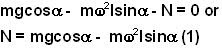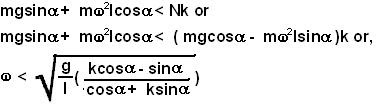## Friday, May 9, 2008

### Irodov Problem 1.146As in problem 1.145, we can replace the cone by its center of mass to answer the question. As seen by an observer in the rotating frame of the CG, there are four forces acting on the cone (and hence the CG), i) the force of gravity mg, ii) the normal reaction N, and iii) the force of friction F opposing the tendency of the cone to slide downwards and iv) the centrifugal forceacting away from the center. As seen from the CG, the CG has no acceleration.

i) Considering the force along the direction of the slope of the cone we have,ii) Considering the direction normal to the slope we have,The maximum force of friction that the surface can offer is F = kN. Thus, for the cone not to slip,